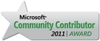# How to update Range of data (Invoices) from other table with condition ?RSS

## 2 replies

Last post Jul 21, 2016 09:52 PM by a.amin

• ### How to update Range of data (Invoices) from other table with condition ?

Jul 18, 2016 11:35 AM|a.amin|LINK

Hi

i try in this query to update some data in table (AZTRH) with some data from table (AZTRD) - they have relation on tr_no field - i try this query that i can update one invoice

```UPDATE  AZTRH
SET     LDisc = ( SELECT    ( SUM(DISC1) + SUM(DISC2) )
FROM      AZTRD
WHERE     TR_TY = 102
AND LOC = 01
AND TR_NO = 215294
) ,
LTAX = ( SELECT ( SUM(TAX1) )
FROM   AZTRD
WHERE  TR_TY = 102
AND LOC = 01
AND TR_NO = 215294
) ,
LTOT = ( (SELECT    SUM(PKPRC * QTY)
FROM      AZTRD
WHERE     TR_NO = 215294
AND TR_TY = 102
AND LOC = 01)
)
WHERE   TR_TY = 102
AND LOC = 01
AND TR_NO = 215294;```

so how can i make it update data i need where tr_no in both table as the same - i they will be in date range  ... ?!

__________________
Best Regards
Eng.Ahmed Amin
ahmed.mo.amin@gmail.com
• ### Re: How to update Range of data (Invoices) from other table with condition ?

Jul 18, 2016 01:09 PM|eralper|LINK

Hi a.amin,

Please check following UPDATE query with SQL CTE expression used for aggration functions like SUM() for values required in update

```;with CTE as (
SELECT
d.TR_TY, d.LOC, d.TR_NO,
LDisc = SUM(DISC1) + SUM(DISC2),
LTAX = SUM(TAX1),
LTOT = SUM(PKPRC * QTY)
FROM AZTRH h
INNER JOIN AZTRD d on d.TR_TY = h.TR_TY and d.LOC = h.LOC and d.TR_NO = h.TR_NO
--WHERE h.TR_TY = 102 and h.LOC = 1 and h.TR_NO = 215294
GROUP BY d.TR_TY, d.LOC, d.TR_NO
)
UPDATE AZTRH
SET
LDisc = CTE.LDisc,
LTAX = CTE.LTAX,
LTOT = CTE.LTOT
FROM AZTRH h
INNER JOIN CTE ON CTE.TR_TY = h.TR_TY and CTE.LOC = h.LOC and CTE.TR_NO = h.TR_NO
```

Please note that I have a WHERE clause in CTE which enables you to limit updated rows on the target table

If you leave as commented, all rows coming from AZTRD table will be updates

I hope it helps

T-SQL programming SQL Server Tutorials
SQL Server 2017• ### Re: How to update Range of data (Invoices) from other table with condition ?

Jul 21, 2016 09:52 PM|a.amin|LINK

#### eralper

Hi a.amin,

Please check following UPDATE query with SQL CTE expression used for aggration functions like SUM() for values required in update

```;with CTE as (
SELECT
d.TR_TY, d.LOC, d.TR_NO,
LDisc = SUM(DISC1) + SUM(DISC2),
LTAX = SUM(TAX1),
LTOT = SUM(PKPRC * QTY)
FROM AZTRH h
INNER JOIN AZTRD d on d.TR_TY = h.TR_TY and d.LOC = h.LOC and d.TR_NO = h.TR_NO
--WHERE h.TR_TY = 102 and h.LOC = 1 and h.TR_NO = 215294
GROUP BY d.TR_TY, d.LOC, d.TR_NO
)
UPDATE AZTRH
SET
LDisc = CTE.LDisc,
LTAX = CTE.LTAX,
LTOT = CTE.LTOT
FROM AZTRH h
INNER JOIN CTE ON CTE.TR_TY = h.TR_TY and CTE.LOC = h.LOC and CTE.TR_NO = h.TR_NO
```

Please note that I have a WHERE clause in CTE which enables you to limit updated rows on the target table

If you leave as commented, all rows coming from AZTRD table will be updates

I hope it helps

Thanks a lot - please if i need to add some other conditions like

`TR_TY = 102 and LOC = 1 `

where i can add them in them (with update part or with select part ?) and if i need to update item price too for each item we it will works ?

it try it as:

```;
WITH    CTE
AS ( SELECT   h.TR_TY ,
h.LOC,
h.TR_NO,
h.ITM_CD,
s.SPRC6 ,
s.TAX1 ,
( CAST(REPLACE(s.DISC1, '%', '') AS FLOAT) / 100 ) AS DISC1 ,
( CAST(REPLACE(s.DISC1_1, '%', '') AS FLOAT) / 100 ) AS DISC1_1 ,
h.QTY ,
h.BONASQTY ,
h.Disc
FROM     AZTRD AS h
INNER JOIN dbo.AZITMS AS s ON s.ITM_CD = h.ITM_CD
WHERE    h.LOC = 01
AND h.TR_TY = 102
AND h.TR_DT BETWEEN '2016-05-18' AND '2016-07-21'
)
UPDATE  AZTRD
SET     PKPRC = ( CTE.SPRC6 - CTE.TAX1 ),
Disc = CAST((((( CTE.SPRC6 - CTE.TAX1 ) * CTE.QTY) * CTE.DISC1) + (( CTE.SPRC6 - CTE.TAX1 ) - (((( CTE.SPRC6 - CTE.TAX1 ) * CTE.QTY) * CTE.DISC1) * CTE.DISC1_1))) AS DECIMAL(18, 4)),
DISC1 = ((( CTE.SPRC6 - CTE.TAX1 ) * CTE.QTY) * CTE.DISC1),
TAX = CTE.TAX1 ,
TAX1 = CTE.TAX1 ,
STAX_VL = ( ( CTE.QTY + CTE.BONASQTY ) * CTE.TAX1 ) ,
STAX_VL1 = ( ( CTE.QTY + CTE.BONASQTY ) * CTE.TAX1 ),
SPRC = CTE.SPRC6 ,
CPRC = CTE.SPRC6 ,
DISC2 = CAST((((( CTE.SPRC6 - CTE.TAX1 ) * CTE.QTY) - ((( CTE.SPRC6 - CTE.TAX1 ) * CTE.QTY) * CTE.DISC1)) * CTE.DISC1_1) AS DECIMAL(18, 4)),
SDISC1 = CTE.DISC1 * 100 ,
SDISC2 = CTE.DISC1_1 * 100
FROM    AZTRD h
INNER JOIN CTE ON CTE.TR_TY = h.TR_TY
AND CTE.LOC = h.LOC
AND CTE.TR_NO = h.TR_NO
AND CTE.ITM_CD = h.ITM_CD```

so are that query will update each row with data i select where ITM_CD is the same and TR_NO is the same too ????!!!!

__________________
Best Regards
Eng.Ahmed Amin
ahmed.mo.amin@gmail.com